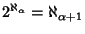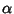## Continuum Hypothesis

The proposal originally made by Georg Cantorthat there is no infinite Set with a Cardinal Number between that of the small'' infinite Set of Integersand the large'' infinite set of Real Numbers(the Continuum''). Symbolically, the continuum hypothesis is that. Gödelshowed that no contradiction would arise if the continuum hypothesis were added to conventional Zermelo-Fraenkel Set Theory. However, using a technique called Forcing, Paul Cohen (1963, 1964) proved that no contradiction would arise if the negation of the continuum hypothesis was added to Set Theory. Together, Gödel's and Cohen's results established that the validity of the continuum hypothesis depends on the version of Set Theory being used, and is therefore Undecidable (assuming the Zermelo-Fraenkel Axioms together with the Axiom of Choice).

Conway and Guy (1996) give a generalized version of the Continuum Hypothesis which is also Undecidable: isfor every?

See also Aleph-0, Aleph-1, Axiom of Choice, Cardinal Number, Continuum, Denumerable Set, Forcing, Hilbert's Problems, Lebesgue Measurability Problem, Undecidable, Zermelo-Fraenkel Axioms, Zermelo-Fraenkel Set Theory

References

Cohen, P. J. The Independence of the Continuum Hypothesis.'' Proc. Nat. Acad. Sci. U. S. A. 50, 1143-1148, 1963.

Cohen, P. J. The Independence of the Continuum Hypothesis. II.'' Proc. Nat. Acad. Sci. U. S. A. 51, 105-110, 1964.

Cohen, P. J. Set Theory and the Continuum Hypothesis. New York: W. A. Benjamin, 1966.

Conway, J. H. and Guy, R. K. The Book of Numbers. New York: Springer-Verlag, p. 282, 1996.

Gödel, K. The Consistency of the Continuum-Hypothesis. Princeton, NJ: Princeton University Press, 1940.

McGough, N. The Continuum Hypothesis.'' http://www.jazzie.com/ii/math/ch/ and http://www.best.com/~ii/math/ch/.